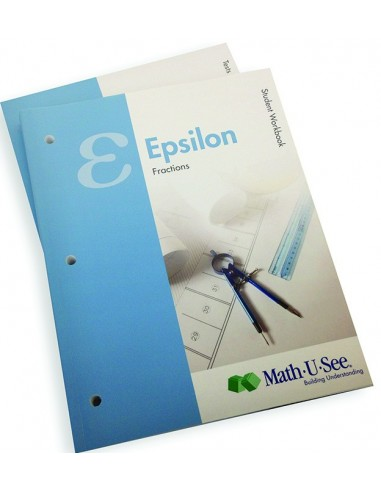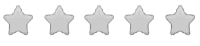See inside this book

# Math-U-See Epsilon Student Pack

\$42.00
No tax
Demme Learning
Math
5
P-MA05-62Leave a Review

#### Description

New! Math-U-See Epsilon - Student Pack

Math-U-See is a complete, skill-based, multi-sensory homeschool math curriculum for grades K-12 that?s designed to teach students skills in a logical sequence.

The Epsilon Student Pack is for those who already have the other required components for the course. It contains the Student Workbook with lesson-by-lesson worksheets, systematic review pages, and Application & Enrichment pages as well as Epsilon Tests.

Now that students have learned basic operations with whole numbers, Epsilon covers these same operations with fractions. Fractions are presented in an intuitive way with visual explanations of equivalent fractions, common denominators, and fractions and numbers larger than 1. Fractions and operations are illustrated using our proprietary Fraction Overlay manipulatives.

Major Concepts and Skills Include:

Recognizing and generating equivalent fractions Understanding addition, subtraction, multiplication, and division of fractions and mixed numbers Fluently adding, subtracting, multiplying, and dividing fractions and mixed numbers

Using multiple strategies to recognize common factors Understanding grouping symbols and their effect on order of operations Interpreting and solving word problems Comparing, and converting decimal fractions Finding the area and circumference of circles Classifying quadrilaterals Representing fractions and fractional measurements on line plots and number lines Using coordinates to represent ordered relationships